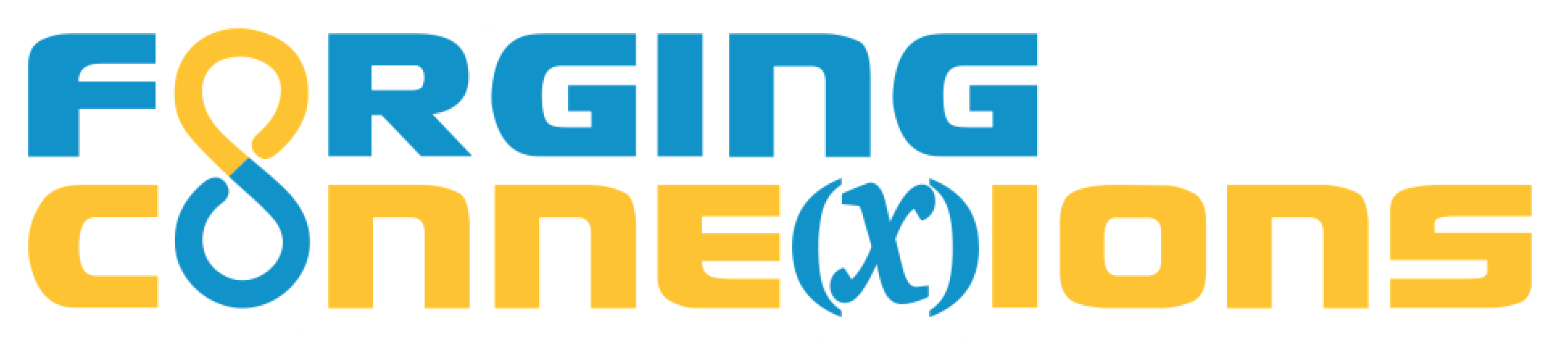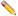The SSS Theorem

In this lesson you’ll prove an important geometric fact: The Side-Side-Side Theorem (SSS).

In Activity 1 you will practice by constructing and proving a simpler theorem.

In Activity 2, you will construct the superposition of SSS triangles, and you will explain how to use the steps of your construction to prove the SSS Theorem.

In Activity 3, you will consider some other cases different from the triangles in Activity 2, and figure out how to make your proof from Activity 2 apply to these cases as well.

1 Prove the Segment Congruence Theorem

Construct and prove the Segment Congruence Theorem: Given AB = CD, the two segments overline(AB) and overline(CD) are congruent.

Remember that congruent figures are figures that can be superposed using and that can be produced by a composition of reflections.

You can rephrase the task: Given AB = CD, use reflections to superpose an image of overline(AB) on overline(CD).

Because this is a proof task, you must be able to explain why each step of your construction works.

Use pages 1, 2, and 3 to develop your proof. Pages 4 and 5 have special cases that you can explore.

On this page you will develop your strategy for superposing one segment on another. The given fact about the lengths of the segments (AB = CD) is already true, so you just need to figure out how to construct the transformations.

• Using the tools provided, transform overline(AB) so that its image is superposed on overline(CD).
• How many perpendicular bisectors, and how many reflections, do you need to superpose these segments?
• Go on to page 2.

On this page the given fact has not yet been applied. You need to figure out when, and why, you need to use the given fact to make your construction work correctly.As you do your construction, when is it most convincing, or most logical, to apply the given fact?

Go on to page 3.

On this page, as you do each step of the construction, describe that step and explain why it works the way it does.Organize your page 3 descriptions and explanations in a clear logical way (paragraph, two-column format, or other format specified by your teacher).

By describing what you did, and explaining why it works, you have proved the Segment Congruence Theorem.

Go on to page 4.

How many reflections did you need to superpose overline(AB) on overline(CD)?

What change could you make so that your proof method from page 3 works normally?

Go on to page 5.

What happens when you try your normal method on this example?

What change could you make so that your proof method from page 3 works normally?

Go on to page 5.

2 Prove the SSS Theorem

Page 1 is for practice. These two triangles already have sides of equal lengths. As the markers show, AB = DE, BC = EF, and CA = FD.

With the sides already equal, here's your first task: Figure out how to use the P. Bisector and Reflect tools to transform tri ABC so that its image is exactly on top of tri DEF.

Page 2 is the real deal. The sides of the two triangles aren't exactly equal in length. What could possibly go wrong?

Here's your page 2 task: (a) Figure out what does go wrong. (b) Use the three Given tools to fix the problems. (c) Explain what went wrong, why it went wrong, and why these three tools fixed it.

Page 3 gives you more practice using the tools that makes the side lengths equal. (These Given tools correspond to the Given facts of the SSS Triangle Congruence Theorem.)

Here is your page 3 task: Figure out how to perform your construction to make your argument for the SSS Triangle Congruence Theorem as easy to understand as you can. In what order would you do the steps to help a friend or a relative understand it?

You may want to consider these questions as you work on page 3: (a) Does it really matter when you use the three Given tools? (b) How does your argument change if you use them all at the beginning, or all at the end? (c) When is the most effective time to use each Given tool to support your argument?

3 The SSS Theorem: Other Cases

Other Cases for the SSS Theorem: In the previous activity, you used three examples to construct and prove the SSS Theorem. In all three of the examples triABC and triDEF were opposite-handed: one clockwise and one counter-clockwise. But what if the two triangles are similarly handed? How can you construct/prove the SSS theorem for similarly-handed triangles?

In this activity you'll do just that, but instead of creating an entirely new proof you'll find a easier way, by using the proof you've already created.Why is it impossible to put two similarly-handed triangles into superposition using the same reflections you used for opposite-handed triangles?What's the simplest way you can transform triABC to make its image opposite-handed compared to triDEF?

On page 1 transform triABC so that the image is opposite-handed to triDEF. Then use the construction from the previous activity to superpose an image of triABC onto triDEF.

Page 2 contains a different example.As you do this construction, describe it and explain why it works. You can simplify your explanation by referring to the proof you created for opposite-handed triangles.

Page 3 contains another special case for you to consider.What can you do with this case to make it easy to prove?

Video hints are not yet available.

5 The Fine Print

Requirements:

These activities require web access using a browser that supports HTML5 and JavaScript. (That means almost any current browser.) No purchase is required, and there’s no advertising anywhere.

Release Information

Update History:

17 Apr 2018: Now includes construction history, more animations, closer connection to proof, etc.